GATE 2012 ECE Video Solutions on EDC (Electronic Devices and Circuits)

1. The I – V characteristics of the diode in the circuit is given below, then the current in the circuit is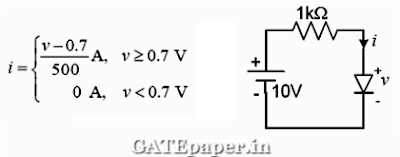a. 10 mA
b. 9.3 mA
c. 6.67 mA
d. 6.2 mA

2. The diodes and capacitors shown in the circuit are ideal. The voltage V(t) across the diode D1 is3. In the circuit shown below, the output expression ‘Y’ is4. In the CMOS circuit shown, electron and hole motilities are equal, and M1 and M2 transistors are equally sized. The device M1 is in the linear region if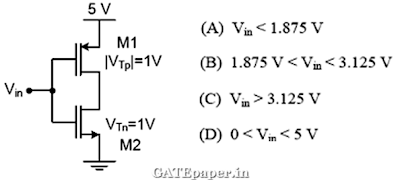5. In the three dimensional view of a silicon N channel MOS transistor shown below, δ = 20 nm. The transistor is of width 1 µm. The depletion width formed at every PN junction is 10 nm. The relative permittivity’s of Si and SiO2 are 11.7 and 3.9 respectively and Ɛ0 = 8.9 X 10-12 F/m.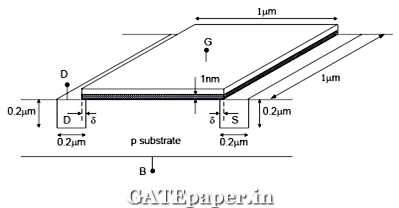i. The gate – source overlap capacitance is approximately
a. 0.7 fF
b. 0.7 pF
c. 0.35 fF
d. 0.24 fF

ii. The source – body capacitance approximately
a. 2 fF
b. 7 fF
c. 2 pF
d. 7 pF

GATE 2014 ECE Video Solutions on EDC (Electronic Devices and Circuits)

Set – 1 (15th February 2014 (Forenoon))

1. When the optical power incident on a photo diode is 10 µW and the responsivity is 0.8 A/W, the photo current generated (in µA) is ____________.

2. In the figure, assume that the forward voltage drops to the PN diode D1 and Schottky diode D2 are 0.7 volts and 0.3 volts respectively. If ON denotes conducting state of the diode and OFF denotes the non conducting state of the diode, then in the circuit,a. Both are ON
b. D1 is ON and D2 is OFF
c. Both are OFF
d. D1 is OFF and D2 is ON

3. If the fixed positive charges are present in the gate oxide of an N channel enhancement type MOSFET, it will lead to
a. a decrease in the threshold voltage
b. channel length modulation
c. an increase in substrate leakage current
d. an increase in accumulation capacitance

4. A good current buffer has
a. Low input impedance and low output impedance
b. Low input impedance and high output impedance
c. high input impedance and low output impedance
d. high input impedance and high output impedance

5. A BJT is biased in forward active mode. Assume VBE = 0.7 volts, KT/q = 25 mV and reverse saturation current IS = 10-13 Amp. The Transconductance of the BJT (in mA/volt) is…..

6. The doping concentration on the P side and N side of a silicon diode are 1x1016 cm-3 and 1x1017 cm-3 respectively. A forward bias of 0.3 volts is applied to the diode. At T = 300oK, the intrinsic carrier concentration of silicon, ni = 1.5 x 1010 cm-3 and KT/q = 26 mV. The electron concentration at the edge of the depletion region on the P side is
a. 2.3 x 109 cm-3
b. 1 x 1016 cm-3
c. 1 x 1017 cm-3
d. 2.25 x 106 cm-3

7. A depletion type N channel MOSFET is biased in its linear region for use as a voltage controlled resistor. Assume threshold voltage VTH = -0.5 volts, VGS = 2.0 volts, VDS = 5 volts, W/L = 100, Cox = 10-8 F/cm2 and µn = 800 cm2/volt-sec. The value of the voltage controlled resistor (in Ω) is …..

SET – 2 (15th February 2014 (Afternoon))

1. A silicon bar is doped with donor impurities ND = 2.25 x 1015 atoms/cm3. Given the intrinsic carrier concentration of silicon at T = 300oK is ni = 1.5 x 1010 cm-3. Assuming complete impurity ionization, the equilibrium electron and hole concentrations are
a. no = 1.5 x 1016 cm-3, p0 = 1.5 x 105 cm-3
b. no = 1.5 x 1010 cm-3, p0 = 1.5 x 1015 cm-3
c. no = 1.5 x 1015 cm-3, p0 = 1.5 x 1010 cm-3
d. no = 1.5 x 1015 cm-3, p0 = 1.5 x 105 cm-3

2. an increase in the base recombination of a BJT will increase
a. the common emitter DC current gain, β
b. the breakdown voltage BVCEO
c. the unity gain cutoff frequency, fτ
d. the Transconductance gm

3. In CMOS technology, shallow P – well or N – well regions can be formed using
a. low pressure chemical vapor deposition
b. low energy sputtering
c. low temperature dry oxidation
d. low energy ion – implantation

4. Assume electron charge q = 1.6 x 10-19C, KT/q = 25 mV and electron mobility µn = 1000 cm2/volt-sec. If the concentration gradient of electrons injected into a P type silicon sample is 1 x 1021 per cm-3, the magnitude of electron diffusion current density (in A/cm2) is ………….

5. Consider an abrupt PN junction (at T = 300oK) shown in the figure. The depletion region width Xn on the N side of the junction is 0.2 µm and the permittivity of silicon (Ԑsi) is 1.044 x 10-12 F/cm. At the junction, the approximate value of the peak electric field (in kV/cm) is………..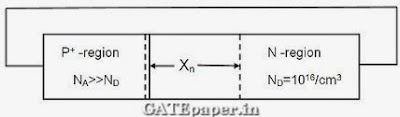6. When a silicon diode having a doping concentration of NA = 9 x 1016 cm-3 on P side and ND = 1 x 1016 cm-3 on N side is reversed biased, the total depletion width is found to be 3 µm. Given that the permittivity of silicon is 1.04 x 10-12 F/cm, the depletion width on the P side and the maximum electric field in the depletion region, respectively, are
a. 2.7 µm and 2.3 x 105 V/cm
b. 0.3 µm and 4.15 x 105 V/cm
c. 0.3 µm and 0.42 x 105 V/cm
d. 2.1 µm and 0.42 x 105 V/cm

7. The diode in the circuit shown, if Von = 0.7 volts but is ideal otherwise. If Vi = 5 sin(ωt) volts, the minimum and maximum values of Vo (in volts) are, respectively,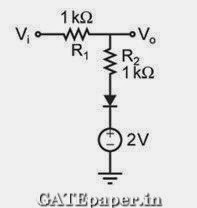a. -5 and 2.7
b. 2.7 and 5
c. -5 and 3.85
d. 1.3 and 5

8. For the N channel MOS transistor shown in the figure, the threshold voltage VTH is 0.8 volts. Neglect channel length modulation effects. When the drain voltage VD = 1.6 volts, the drain current ID was found to be 0.5 mA. If VD is adjusted to be 2 volts by changing the values of R and VDD, the new value of ID (in mA) is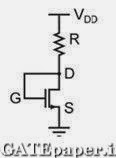a. 0.625
b. 0.75
c. 1.125
d. 1.5

9. For the MOSFETs shown in the figure, the threshold voltage |Vt| = 2 volts and K = 0.5µcox(W/L) = 0.1 mA/V2. The value of ID (in mA) is …………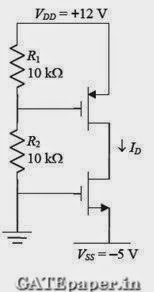SET – 3 (16th February 2014 (Forenoon))

1. In MOSFET fabrication, the channel length is defined during the process of
a. Isolation oxide growth
b. Channel stop implantation
c. Poly-silicon gate patterning

2. A thin P type silicon sample is uniformly illuminated with light which generates excess carriers. The recombination rate is directly proportional to
a. The minority carrier mobility
b. The minority carrier recombination lifetime
c. The majority carrier concentration
d. The excess minority carrier concentration

3. At T = 300oK, the hole mobility of a semiconductor µp = 500 cm2/volt-sec and KT/q = 26 mV. The hole diffusion constant Dp in cm2/sec is ………

4. In the circuit shown, the PNP transistor has |VBE| = 0.7 volts and β = 50. Assume that RB = 100 KΩ. For Vo to be 5 volts, the value of RC (in KΩ) is ……..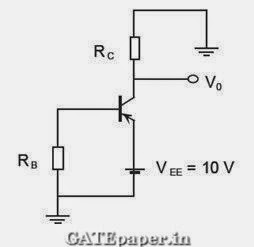5. The donor and acceptor impurities in an abrupt junction silicon diode are 1 x 1016 cm-3 and 5 x 1018 cm-3, respectively. Assume that the intrinsic carrier concentration in silicon ni = 1.5 x 1010 cm-3 at 300oK, KT/q = 26 mV and the permittivity of silicon Ԑsi = 1.04 x 10-12 F/cm. The built in potential and the depletion width of the diode under thermal equilibrium conditions, respectively are
a. 0.7 volts and 1 x 10-4 cm
b. 0.86 volts and 1 x 10-4 cm
c. 0.7 volts and 3.3 x 10-5 cm
d. 0.86 volts and 3.3 x 10-5 cm

6. The slope of the ID vs. VGS curve of an N channel MOSFET in linear region is 10-3-1 at VDS = 0.1 volts. For the same device, neglecting channel length modulation, the slope of the √ID vs. VGS curve (in √A/V) under saturation regime is approximately…………….

7. An ideal MOS capacitor has boron doping concentration of 1015 cm-3 in the substrate. When a gate voltage is applied, a depletion region of width 0.5 µm is formed with a surface (channel) potential of 0.2 volts. Given that Ԑo = 8.854 x 10-14 F/cm and the relative permittivity’s of silicon and silicon dioxide are 12 and 4 respectively. The peak electric field (in V/µm) in the oxide region is ……………..

8. In the circuit shown, the silicon BJT has β = 50. Assume VBE = 0.7 volts and VCEsat = 0.2 volts. Which one of the following statements is correct?a. For RC = 1 kΩ, the BJT operates in the saturation region
b. For RC = 3 kΩ, the BJT operates in the saturation region
c. For RC = 20 kΩ, the BJT operates in the cutoff region
d. For RC = 20 kΩ, the BJT operates in the linear region

9. For the MOSFET M1 shown in the figure, assume W/L = 2, VDD = 2.0 volts, µncox = 100 µA/V2 and VTH = 0.5 volts. The transistor M1 switches from saturation region to linear region when Vin (in volts) is …………..SET - 4 (16th February 2014 (Afternoon))

1. At T = 300oK, the band gap and the intrinsic carrier concentration of GaAs are 1.42 eV and 106 cm-3 respectively. In order to generate electron hole pairs in GaAs, which one of the wavelength (λc) ranges of incident radiation, is most suitable? (Given that Plank’s constant = 6.62 x 10-34 J-sec, velocity of light is 3 x 1010 cm/s and charge of electron is 1.6 x 10-19C)
a. 0.42 µm < λc < 0.87 µm
b. 0.87 µm < λc < 1.42 µm
c. 1.42 µm < λc < 1.62 µm
d. 1.62 µm < λc < 6.62 µm

2. In the figure, ln(ρi) is plotted as a function of 1/T, where ρi is the intrinsic resistivity of silicon, T is the temperature, and the plot is almost linear. The slope of the line can be used to estimatea. Band gap energy of silicon
b. Sum of electron and hole mobility in silicon
c. Reciprocal of the sum of electron and hole mobility in silicon
d. Intrinsic carrier concentration of silicon

3. The cutoff wavelength (in µm) of light that can be used for intrinsic excitation of a semiconductor material of band gap Eg = 1.1 eV is …………..

4. If the emitter resistance in a common emitter voltage amplifier is not bypassed, it will
a. Reduce both the voltage gain and the input impedance
b. Reduce the voltage gain and increase the input impedance
c. Increase the voltage gain and reduce the input impedance
d. Increase both the voltage gain and the input impedance

5. Consider a silicon sample doped with ND = 1 x 1015 /cm3 donor atoms. Assume that the intrinsic carrier concentration ni = 1.5 x 1010 cm-3. If the sample is additionally doped with NA = 1 x 1018 cm-3 acceptor atoms, the approximate number of electrons /cm3 in the sample, at T = 300oK, will be…………

6. Consider two BJTs biased at the same collector current with area A1 = 0.2 µm x 0.2 µm and A2 = 300 µm x 300 µm. assuming that all other device parameters are identical, KT/q = 26 mV, the intrinsic carrier concentration is 1 x 1010 cm-3, and q = 1.6 x 10-19C, the difference between the base emitter voltages (in mV) of the two BJTs (i.e. VBE1 – VBE2) is …………….

7. An N type semiconductor having uniform doping is biased as shown in the figure.8. A BJT in a common base configuration is used to amplify a signal received by a 50Ω antenna. Assume KT/q = 25 mV, the value of collector bias current (in mA) required to match the input impedance of the amplifier to the impedance of the antenna is ………..

GATE 2013 ECE Video Solutions on EDC (Electronic Devices and Circuits)

1. In IC technology, Dry oxidation(using dry oxygen) as compared to Wet oxidation (using stream or water vapor) produces………..
a. Superior quality oxide with a higher growth rate
b. Inferior quality oxide with a higher growth rate
c. Inferior quality oxide with a lower growth rate
d. Superior quality oxide with a lower growth rate

2. For a forward biased PN junction diode, the sequence of events that best describes the mechanism of current flow is
a. Injection and subsequent diffusion and recombination of minority carriers
b. Injection and subsequent drift and generation of minority carriers
c. Extraction and subsequent diffusion and generation of minority carriers
d. Extraction and subsequent drift and recombination of minority carriers

3. In MOSFET operating in saturation region, the channel length modulation effect causes
a. An increase in gate source capacitance
b. A decrease in Transconductance
c. A decrease in unity gain bandwidth product
d. A decrease in output resistance

4. In the circuit shown below, the knee current of ideal zener diode is 10 mA. To maintain 5 volts across the load RL, the minimum value of RL in Ω’s and the minimum power rating of the zener diode in mW respectively area. 125 and 125
b. 125 and 250
c. 250 and 125
d. 250 and 250

5. In the circuit shown below, the silicon NPN transistor Q has a very high value of β. The required value of R2 in KΩ to produce IC = 1 mA is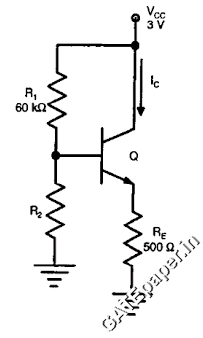a. 20
b. 30
c. 40
d. 50

GATE 2011 ECE Video Solutions on EDC (Electronic Devices and Circuits)

1. A silicon PN junction is forward biased with a constant current at room temperature. When the temperature is increased by 10oC, the forward bias voltage across the PN junction
a. Increases by 60 mV
b. Decreases by 60 mV
c. Increases by 25 mV
d. Decreases by 25 mV

2. Drift current in semiconductors depends upon
a. Only the electric field
b. Only the carrier concentration gradient
c. Both the electric field and the carrier concentration
d. Both the electric field and the carrier concentration gradient

3. A Zener diode when used in voltage stabilization circuits, is biased in
a. Reverse bias region below the breakdown voltage
b. Reverse breakdown region
c. Forward bias region
d. Forward bias constant current mode

4. For the BJT Q1 in the circuit shown, β = ∞, VBEon = 0.7 volts, VCEsat = 0.7 volts. The switch is initially closed. At time t=0, the switch is opened. The time at which Q1 leaves the active region is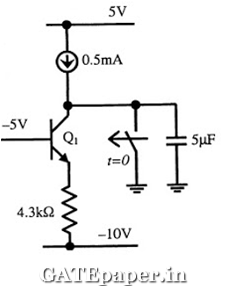a. 10 ms
b. 25 ms
c. 50 ms
d. 100 ms

5. In the circuit shown below, for the MOS transistors, µncox = 100 µA/V2 and the threshold voltage VT = 1 volt. The voltage VX at the source of the upper transistor isa. 1 volt
b. 2 volts
c. 3 volts
d. 0.367 volts

6. For a BJT, the common base current gain α = 0.98 and the collector base junction reverse bias saturation current, ICO = 0.6 µA. This BJT is connected in the common emitter mode and operated in the active region with a base current (IB) of 20 µA. The collector current IC for this mode of operation is
a. 0.98 mA
b. 0.99 mA
c. 1.0 mA
d. 1.01 mA

7. Common Data Question:
The channel resistance of an N channel JFET is shown below is 600 Ω, when the full channel thickness (tOX) of 10 µm is available for conduction. The built in voltage of the gate P+N junction is (Vbi) is -1 volts. When the gate to source voltage (VGS) is 0 volts, the channel is depleted by 1 µm on each side due to the built in voltage and hence the thickness available for conduction is only 8 µm.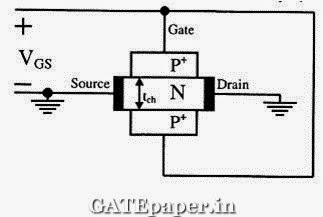i. The channel resistance when VGS = 0 volts is
a. 480 Ω
b. 600 Ω
c. 750 Ω
d. 1000 Ω

ii. The channel resistance when VGS = -3 volt is
a. 360 Ω
b. 917 Ω
c. 1000 Ω
d. 3000 Ω

GATE 2010 ECE Video Solutions on EDC (Electronic Devices and Circuits)

1. At room temperature, a possible value for the mobility of electrons in the inversion layer of a silicon N channel MOSFET is (in cm2/volt-sec)
a. 450
b. 1350
c. 1800
d. 3600

2. The gate oxide in a CMOS process is preferably grown using
a. Wet oxidation
b. Dry oxidation
c. Epitaxial deposition
d. Ion implantation

3. In a uniformly doped BJT, assume that NE, NB and NC are the emitter, base and collector doping in atoms/cm3 respectively. If the emitter injection efficiency of the BJT is close to unity, which of the following conditions is TRUE?
a. NE = NB = NC
b. NE >> NB and NB > NC
c. NE = NB and NB < NC
d. NE < NB < NC

4. Compared to a PN junction with ND=NA=1014 per cm3, which one of the following statements is TRUE for a PN junction with NA=ND=1020 per cm3?
a. Reverse breakdown voltage is lower and depletion capacitance is lower
b. Reverse breakdown voltage is higher and depletion capacitance is lower
c. Reverse breakdown voltage is lower and depletion capacitance is higher
d. Reverse breakdown voltage is higher and depletion capacitance is higher

The silicon sample with unit cross sectional area shown below is in thermal equilibrium. The following information is given:
T = 300oK
Electron charge = 1.6x10-19 C
Thermal voltage = 26 mV
Electron mobility = 1350 cm2/volt-sec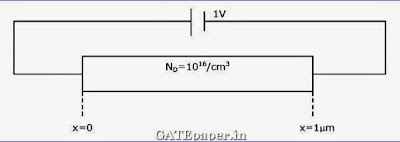i. The magnitude of the electric field at x = 0.5 µm is
a. 1 KV/cm
b. 5 KV/cm
c. 10 KV/cm
d. 26 KV/cm

ii. The magnitude of the electron drift current density at x = 0.5 µm is
a. 2.16x104 A/cm2
b. 1.08x104 A/cm2
c. 4.32x103 A/cm2
d. 6.48x102 A/cm2

GATE 2009 ECE Video Solutions on EDC (Electronic Devices and Circuits)

1. In an N type silicon crystal at room temperature, which of the following can have a concentration of 4x1019 cm-3?
a. Silicon atoms
b. Holes
c. Dopant atoms
d. Valence electrons

2. The full forms of the abbreviations TTL and CMOS in reference to logic families are
a. Triple Transistor Logic and Chip Metal Oxide semiconductor
b. Tristate Transistor Logic and Chip Metal Oxide semiconductor
c. Transistor Transistor Logic and Complementary Metal Oxide semiconductor
d. Tristate Transistor Logic and Complementary Metal Oxide semiconductor

3. The ratio of the mobility to the diffusion coefficient in a semiconductor has the units
a. V-1
b. cm. V-1
c. V. cm-1
d. V.sec

4. In the circuit below, the diode is ideal. The voltage V is given by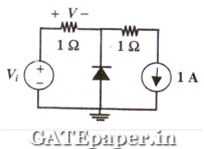a. Min(Vi,1)
b. Max(Vi,1)
c. Min(-Vi,1)
d. Max(-Vi,1)

5. Consider the following two statements about the internal conditions in an N channel MOSFET operating in the active region.
S1: the inversion charge decreases from source to drain
S2: the channel potential increases from source to drain
Which of the following is correct?
a. Both are TRUE
b. Both are FALSE
c. Both are TRUE, but S2 is not a reason for S1
d. Both are TRUE, and S2 is a reason for S1.

6. Common Data Question:
Consider a silicon PN junction at room temperature having the following parameters:
Doping on the N side = 1x1017 cm-3
Depletion width on N side = 0.1 µm
Depletion width on the P side = 1 µm
Intrinsic carrier concentration = 1.4x1010 cm-3
Thermal voltage = 26 mV
Permittivity of free space = 8.85x10-14 F/cm
Dielectric constant of silicon = 12

i. The built in potential of the junction is
a. 0.70 volts
b. 0.76 volts
c. 0.82 volts
d. Cannot be estimated from given data

ii. The peak electric field in the device is
a. 0.15 Mvolts/cm, directed from P region to N region
b. 0.15 Mvolts/cm, directed from N region to P region
c. 1.80 Mvolts/cm, directed from P region to N region
d. 1.80 Mvolts/cm, directed from N region to P region

Consider the CMOS circuit shown, where the gate voltage VG of the N channel MOSFET is increased from zero, while the gate voltage of the P channel MOSFET is kept constant at 3 volts. Assume that, for both transistors, the magnitude of the threshold voltage is 1 volts and the product of the transconductance parameter and the (W/L) ratio i.e. the quantity µcox(W/L) is 1 mA/V2.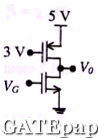i. For small increase in VG beyond 1 volt, which of the following gives the correct description of the region of operation of each MOSFET?
a. Both are in saturation region
b. Both are in triode region
c. NMOS is in triode region and PMOS is in saturation region
d. NMOS is in saturation region and PMOS is in triode region

ii. Estimate the output voltage, Vo for VG = 1.5 volts. (Hint: use the appropriate current voltage equation for each MOSFET, based on the answer to above question)GATE 2008 ECE Video Solutions on EDC (Electronic Devices and Circuits)

1. Which of the following is NOT associated with a PN junction?
a. Junction capacitance
b. Charge storage capacitance
c. Depletion capacitance
d. Channel length modulation

2. Which of the following is TRUE?
a. A silicon wafer heavily doped with boron is a P+ substrate
b. A silicon wafer lightly doped with boron is a P+ substrate
c. A silicon wafer heavily doped with Arsenic is a P+ substrate
d. A silicon wafer lightly doped with Arsenic is a P+ substrate

3. In the following limiter circuit, an input voltage Vi = 10sin100πt applied. Assume that the diode drop is 0.7 volts when it is forward biased. The zener breakdown voltage is 6.8 volts.
The maximum and minimum values of the output voltage respectively area. 6.1 volts, -0.7 volts
b. 0.7 volts, -7.5 volts
c. 7.5 volts, -0.7 volts
d. 7.5 volts, -7.5 volts

4. A silicon wafer has 100nm of oxide on it and is inserted in a furnace at a temperature above 1000oC for further oxidation in dry oxygen. The oxidation rate
a. Is independent of current oxide thickness and temperature
b. Is independent of current oxide thickness but depends on temperature
c. Slows down as the oxide grows
d. Is zero as the existing oxide prevents further oxidation

5. The drain current of a MOSFET in saturation is given by ID = K(VGS-VT)2, where K is a constant. The magnitude of the Transconductance gm is

6. For the circuit shown in the following figure, transistors M1 and M2 are identical NMOS transistors. Assume that M2 is in saturation and the output is unloaded. The IX is related to Ibias as7. The measured Transconductance gm of an NMOS transistor operating in the linear region is plotted against the gate voltage VG at constant drain voltage VD. Which of the following figures represents the expected dependence of gm on VG?8. Two identical NMOS transistors M1 and M2 are connected as shown below. Vbias is chosen so that both transistors are in saturation. The equivalent gm of the pair is defined to be dIout / dVi at constant Vout, is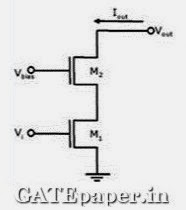a. The sum of individual gm’s of the transistors
b. The product of individual gm’s of the transistors
c. Nearly equal to the gm of M1
d. Nearly equal to gm1/gm2 of M2

9. Silicon is doped with boron to a concentration of 4x1017atoms/cm3. Assuming the intrinsic carrier concentration of silicon to be 1.5x1010 cm-3 and the value of KT/q to be 25 mV at 300oK. Compared to undoped silicon, the Fermi level of doped silicon
a. Goes down by 0.13 eV
b. Goes up by 0.13 eV
c. Goes down by 0.427 eV
d. Goes up by 0.427 eV

10. The cross section of a JFET is shown in the figure. Let VG be -2 volts and VP be the initial pinch off voltage. If the width W is doubled (with other geometric parameters and doping levels remaining the same), then the ratio between the mutual Transconductance of the initial and the modified JFET is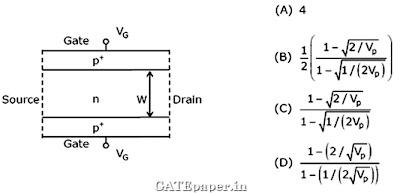11. Consider the following assertions.
S1: For zener effect to occur, a very abrupt junction is required
S2: For quantum tunneling to occur, a very narrow energy barrier is required.
Which of the following is correct?
a. Only S2 is true
b. Both are true but S2 is not a reason for S1
c. Both are true but S2 is a reason for S1
d. Both are false

GATE 2007 ECE Video Solutions on EDC (Electronic Devices and Circuits)

1. The electron and hole concentrations in an intrinsic semiconductor are ni per cm3 at 300oK. Now if acceptor impurities are introduced with a concentration of NA per cm3 (where NA > ni), then electron concentration per cm3 at 300oK will be
a. ni
b. ni + NA
c. NA – ni
d. ni2 / NA

2. In a P+N junction diode under reverse bias, the magnitude of electric field is maximum at
a. The edge of the depletion region on P side
b. The edge of the depletion region on N side
c. The P+N junction
d. The center of the depletion region on the N side

3. The correct full wave rectifier circuit is :4. A P+N junction has a built in potential of 0.8 volts. The depletion layer width at a reverse bias of 1.2 volts is 2 µm. For a reverse bias of 7.2 volts, the depletion layer width will be
a. 4 µm
b. 4.9µm
c. 8 µm
d. 12 µm

5. Group I lists four types of PN junction diodes. Match each device in Group I with one of the option in Group II to indicate the bias condition of that device in its normal mode of operation.6. The DC current gain (β) of a BJT is 50. Assuming that the emitter injection efficiency is 0.995, the base transport factor is:
a. 0.980
b. 0.985
c. 0.990
d. 0.995

7. Group I lists four different semiconductor devices. Match each device in Group I with its characteristic property in Group II.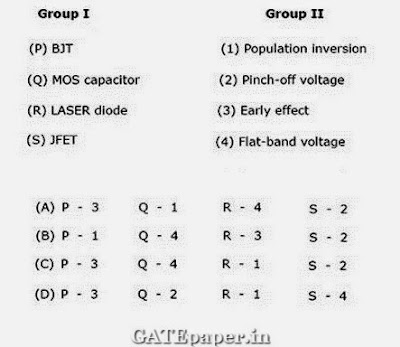8. For the BJT circuit shown, assume that the β of the transistor is very large and VBE is 0.7 volts. The mode of operation of the BJT isa. Cut off
b. Saturation
c. Normal active
d. Inverse active

9. In the CMOS inverter circuit shown, if the Transconductance parameters of the NMOS and PMOS transistors are Kn = Kp = µnCox(Wn/Ln) = µpCox(Wp/Lp) = 40 µA/V2 and their threshold voltages are VTn = |VTp| = 1 volt, the current I isa. 0 Amp
b. 25 µA
c. 45 µA
d. 90 µA

10. For the zener diode shown in the figure, the zener voltage at knee is 7 volts, the knee current is negligible and the zener dynamic resistance is 10 Ω. If the input voltage (Vi) range is 10 to 16 volts, the output voltage (Vo) ranges from11. The figure shows the high frequency capacitance – voltage (C – V) characteristics of MOS capacitor having an area of 1x10-4 cm2. Assume that the permittivity of silicon and SiO2 are 1x10-12 and 3.5x10-13 F/cm respectively.i. The gate oxide thickness in the MOS capacitor is
a. 50 nm
b. 143 nm
c. 350 nm
d. 1 µm

ii. The maximum depletion layer width in silicon is
a. 0.143 µm
b. 0.857 µm
c. 1 µm
d. 1.143 µm

iii. Consider the following statements about the C – V characteristics plot :
S1: The MOS capacitor has an N type substrate
S2: If the positive charges are introduced in the oxide, the C – V plot will shift to the left.
Then which one of the following is TRUE .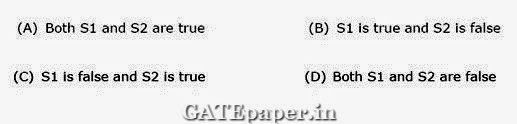GATE 2006 ECE Video Solutions on EDC (Electronic Devices and Circuits)

1. The values of voltage across a tunnel diode (VD) corresponding to peak and valley currents are VP and VV respectively. The range of tunnel diode voltage VD for which the slope of its I-V characteristics is negative would be
a. VD < 0
b. 0 ≤ VD ≤ VP
c. VP ≤ VD ≤ VV
d. VD ≥ VV

2. The concentration of minority carriers in an extrinsic semiconductor under equilibrium is
a. Directly proportional to the doping concentration
b. Inversely proportional to the doping concentration
c. Directly proportional to the intrinsic concentration
d. Inversely proportional to the intrinsic concentration

3. Under low level injection assumption, the injected minority carrier current for an extrinsic semiconductor is essentially the
a. Diffusion current
b. Drift current
c. Recombination current
d. Induced current

4. The phenomenon known as “early effect” in a BJT refers to a reduction of the effective base width caused by
a. Electron – hole recombination at the base
b. The reverse biasing of the base collector junction
c. The forward biasing of emitter base junction
d. The early removal of stored base charge during saturation to cutoff switching

5. An N-channel depletion MOSFET has the following two points on its ID verses VGS curve are
(i) VGS = 0 at ID = 12 mA and
(ii) VGS = -6 volts at ID = 0 mA
Which of the following Q-points will give the highest Transconductance gain for small signals?
a. VGS = -6 volts
b. VGS = -3 volts
c. VGS = 0 volts
d. VGS = 3 volts

6. In the circuit shown below, the switch was connected to position 1 at t < 0, and at t = 0, it is changed to position 2. Assume that the diode has zero voltage drop and a storage time tS. For 0<t<tS, the voltage across the 1 KΩ resistor (VR) in volts is7. The majority carriers in an N-type semiconductor have an average drift velocity V in a direction perpendicular to a uniform magnetic field B. The electric field E induced due to hall effect acts in the direction
a. V x B
b. B x V
c. along V
d. opposite to V

8. Find the correct match between Group 1 and Group 2 :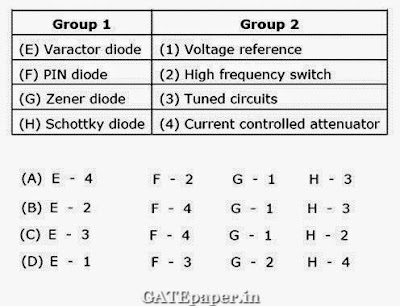9. A heavily doped N-type semiconductor has the following data:
Hole – electron mobility ratio : 0.4
Doping concentration : 4.2 x 108 /m3
Intrinsic concentration : 1.5 x 104 /m3
The ratio of conductance of the N-type semiconductor to that of the intrinsic semiconductor of same material and at the same temperature is given by
a. 0.00005
b. 2000
c. 10000
d. 20000

10. For the circuit shown below, assume the zener diode is ideal with a breakdown voltage of 6 volts. The waveform observed across R is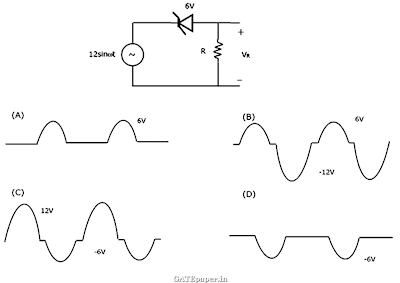GATE 2005 ECE Video Solutions on EDC (Electronic Devices and Circuits)

1. The band gap of silicon at room temperature is
a. 1.3 eV
b. 0.7 eV
c. 1.1 eV
d. 1.4 eV

2. A silicon PN junction at a temperature of 20oC has a reverse saturation current of 10 pA. The reverse saturation current at 40oC for the same bias is approximately
a. 30 pA
b. 40 pA
c. 50 pA
d. 60 pA

3. The primary reason for the widespread use of silicon in semiconductor device technology is
a. Abundance of silicon on the surface of the earth
b. Larger band gap of silicon in comparison to germanium
c. Favorable properties of silicon – dioxide (SiO2)
d. Lower melting point

4. A silicon sample A is doped with 1018 atoms/cm3 of boron. Another sample B of identical dimensions is doped with 1018 atoms/cm3 of phosphorous. The ratio of electron to hole mobility is 3. The ratio of conductivity of the sample A to B is
a. 3
b. 1/3
c. 2/3
d. 3/2

5. A silicon PN junction diode under reverse bias has depletion region of width of 10 µm. The relative permittivity of silicon (Ԑr) is 11.7 and the permittivity of free space (Ԑo) is 8.85 x 10-12 F/m. The depletion capacitance of the diode per square meter is
a. 100 µF
b. 10 µF
c. 1 µF
d. 20 µF

6. For NPN transistor connected as shown in figure, VBE = 0.7 volts. Given that the reverse saturation current of the junction at room temperature is 10-13 Amp, then the emitter current is7. An N-channel MOSFET and its transfer curve is shown in figure, then the threshold voltage is8. The zener diode in the regulator circuit shown has a zener voltage of 5.8 volts and a zener knee current of 0.5 mA. The maximum load current drawn from this circuit ensuring proper functioning over the input voltage range between 20 and 30 volts is9. Both transistors T1 and T2 in figure have a threshold voltage of 1 volt. The device parameters K1 and K2 of T1 and T2 are 36 µA/v2 and 9 µA/v2 respectively. The output voltage Vo is10. A MOS capacitor made using P type substrate is in the accumulation mode. The dominant charge is due to the presence of
a. Holes
b. Electrons
c. Positively charged ions
d. Negatively charged ions

GATE 2004 ECE Video Solutions on EDC (Electronic Devices and Circuits)

1. The impurity commonly used for realizing the base region of a silicon NPN transistor is
a. Gallium
b. Indium
c. Boron
d. Phosphorous

2. If for a silicon NPN transistor, the base to emitter voltage (VBE) is 0.7 volts and collector to base voltage (VCB) is 0.2 volts, then the transistor is operating in the
a. Normal active mode
b. Saturation mode
c. Inverse active mode
d. Cutoff mode

3. Consider the following statements S1 and S2.
S1: the β of a BJT reduces if the base width is increased
S2: the β of a BJT increases if the doping concentration in the base is increased
Which of the following is correct?
a. S1 is FALSE and S2 is TRUE
b. Both S1 and S2 are TRUE
c. Both S1 and S2 are FALSE
d. S1 is TRUE and S2 is FALSE

4. Assuming VCEsat = 0.2 volts and β = 50, the minimum base current (IBmin) required to drive the transistor in figure to saturation is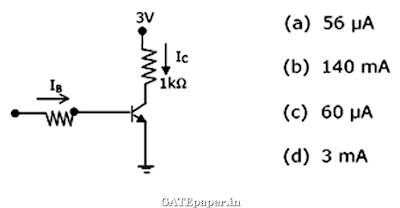5. The given figure is the voltage transfer characteristic of6. In abrupt PN junction, the doping concentrations on the P side and N side are NA = 9 x 1016 cm-3 and ND = 1 x 1016 cm-3 respectively. The PN junction is reverse biased and the total depletion width is 3 µm. The depletion width on the P side is
a. 2.7 µm
b. 0.3 µm
c. 2.25 µm
d. 0.75 µm

7. The resistivity of a uniformly doped N type silicon sample is 0.5 Ω-cm. If the electron mobility (µn) is 1250 cm2/V-sec and the charge of an electron is 1.6 x 10-19 coulomb, then the donor impurity concentration (ND) in the sample is
a. 2 x 1016 / cm3
b. 1 x 1016 / cm3
c. 2.5 x 1015 / cm3
d. 2 x 1015 / cm3

8. Consider an abrupt PN junction. Let Vbi be the built in potential of this junction and VR be the applied reverse bias. If the junction capacitance (CJ) is 1 pF for Vbi + VR = 1 volt, then for Vbi + VR = 4 volts, the value of CJ will be
a. 4 pF
b. 2 pF
c. 0.25 pF
d. 0.75 pF

9. Consider the following statements S1 and S2.
S1: the threshold voltage (VT) of a MOS capacitor decreases with increase in gate oxide thickness
S2: the threshold voltage (VT) of a MOS capacitor decreases with increase in substrate doping concentration.
Which of the following is correct?
a. S1 is FALSE and S2 is TRUE
b. Both S1 and S2 are TRUE
c. S1 is TRUE and S2 is FALSE
d. Both S1 and S2 are FALSE

10. The drain of an N channel MOSFET is shorted to the gate so that VGS = VDS. The threshold voltage (VT) of MOSFET is 1 volt. If the drain current (ID) is 1 mA for VGS = 2 volts, then for VGS = 3 volts, ID is
a. 2 mA
b. 3 mA
c. 9 mA
d. 4 mA

11. The longest wavelength that can be absorbed by silicon, which has the band gap of 1.12 eV is 1.1 µm. If the longest wavelength that can be absorbed by another material is 0.87 µm, then the band gap of this material is
a. 1.416 eV
b. 0.886 eV
c. 0.854 eV
d. 0.706 eV

12. The neutral base width of a bipolar transistor biased in the active region is 0.5 µm. The maximum electron concentration and the diffusion constant in the base are 1014 cm-3 and Dn = 25 cm2/sec respectively. Assuming negligible recombination in the base, the collector current density is
a. 800 A/cm2
b. 8 A/cm2
c. 200 A/cm2
d. 2 A/cm2

13. Assuming that the β of the transistor is extremely large and VBE = 0.7 volts, IC and VCE in the circuit shown are14. In the voltage regulator shown, the load current can vary from 100 mA to 500 mA. Assuming that the zener diode is ideal (i.e. the zener knee current is negligibly small and zener resistance is zero in the breakdown region), the value of R isGATE 2003 ECE Video Solutions on EDC (Electronic Devices and Circuits)

1. N – Type silicon is obtained by doping silicon with
a. Germanium
b. Aluminum
c. Boron
d. Phosphorous

2. The band gap of silicon at 3000K is
a. 1.36 eV
b. 1.10 eV
c. 0.80 eV
d. 0.67 eV

3. The intrinsic carrier concentration of silicon sample at 300oK is 1.5 x 1016 m-3. If after doping, the number of majority carriers is 5 x 1020 m-3, the minority carrier density is
a. 4.5 x 1011 / m3
b. 3.3 x 104 / m3
c. 5 x 1020 / m3
d. 3 x 10-5 / m3

4. Choose proper substitutes for X and Y to make the following statement correct.
Tunnel diode and Avalanche photo diode are operated in X bias and Y bias respectively.
a. X: Revere , Y: Reverse
b. X: Revere, Y: Forward
c. X: Forward, Y: Reverse
d. X: Forward, Y: Forward

5. For an N channel type MOSFET, if the source is connected at a higher potential than that of the bulk (i.e. VSB > 0 volts), the threshold voltage VT of the MOSFET will
a. Remain unchanged
b. Decrease
c. Change polarity
d. Increase

6. An N type silicon bar 0.1 cm long and µm2 in cross sectional area has a majority carrier concentration of 5 x 1020 m-3 and the carrier mobility is 0.13 m2/V-sec at 300oK. If the charge of an electron is 1.6 x 10-19 coulomb, then the resistance of the bar is
a. 106
b. 104
c. 10-1
d. 10-4

7. The electron concentration in a sample of uniformly doped N type silicon at 300oK varies linearly from 1017 cm-3 at x = 0 µm to 6 x 1016 cm-3 at x = 2 µm. Assume a situation that electrons are supplied to keep this concentration gradient constant with time. If electronic charge is 1.6 x 10-19 coulomb and the diffusion constant Dn = 35 cm2/sec, the current density in the silicon, if no electric field is present is
a. Zero
b. -112 A/cm2
c. +1120 A/cm2
d. -1120 A/cm2

8. Match items in Group 1 with items in Group 2, most suitably,9. At 300oK, for a diode current of 1 mA, a certain Germanium diode requires a forward bias of 0.1435 volts, where as a certain Silicon diode requires a forward bias of 0.718 volts. Under the conditions stated above, the closest approximation of the ratio of reverse saturation current in Ge to that of Si diode is
a. 1
b. 5
c. 4 x 103
d. 8 x 103

10. A particular green LED emits light of wavelength 5490 Ao. The energy band gap of the semiconductor material used there is (Plank’s constant = 6.626 x 1034 J-Sec)
a. 2.26 eV
b. 1.98 eV
c. 1.17 eV
d. 0.74 eV

11. When the gate to source voltage (VGS) of a MOSFET with threshold voltage of 400 mV. The drain current observed is 1 mA. Neglecting the channel length modulation effect, and assuming that the MOSFET is operating at saturation, the drain current for an applied VGS of 1400 mV is
a. 0.5 mA
b. 2.0 mA
c. 3.5 mA
d. 4.0 mA

12. If P is passivation, Q is n-well implant, R is metallization and S is source/drain diffusion, then the order in which they are carried out in a standard n-well CMOS fabrication process is
a. P-Q-R-S
b. Q-S-R-P
c. R-P-S-Q
d. S-R-Q-P

13. The action of a JFET in its equivalent circuit can best be represented as a
a. Current controlled current source
b. Current controlled voltage source
c. Voltage controlled current source
d. Voltage controlled voltage source